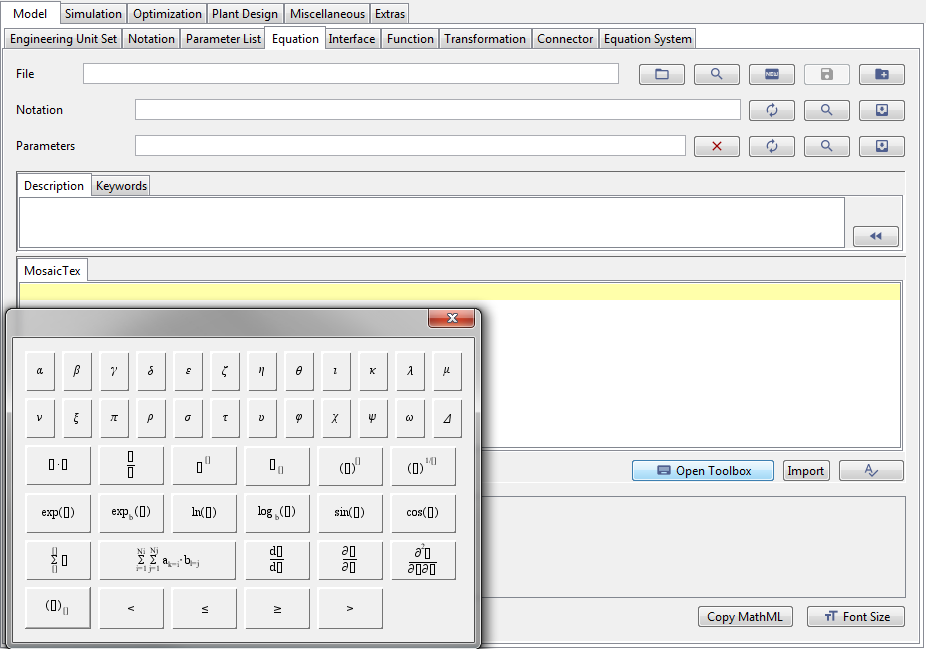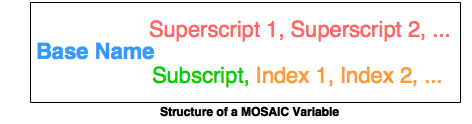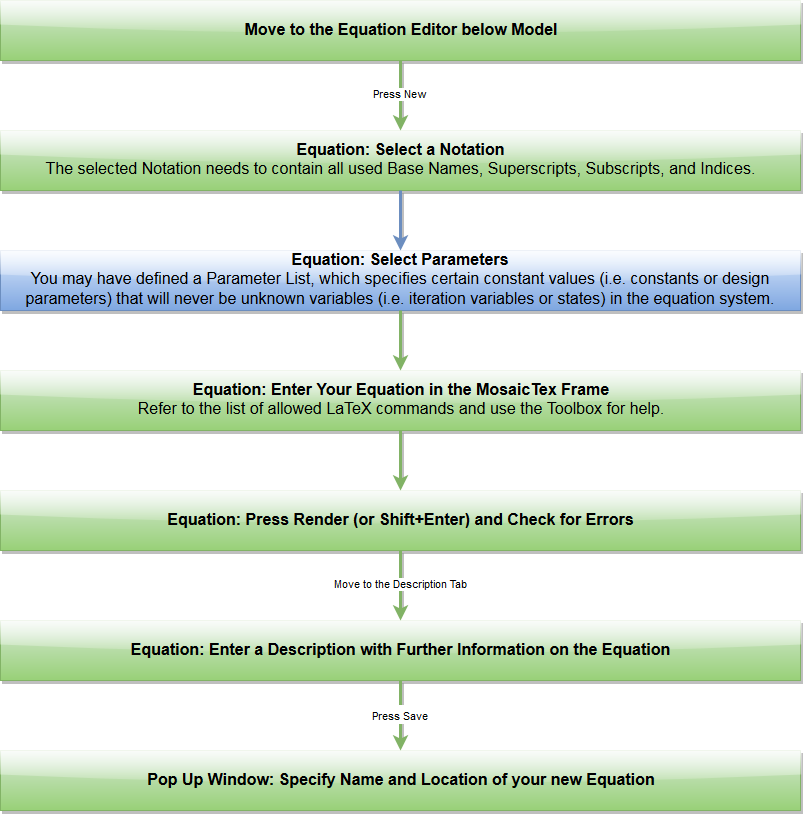# The MOSAICmodeling Workflow – Equation

Basic MOSAICmodeling models consist of Equations with a single Notation, which are then joined together to form an Equation System, which can be simulated or evaluated.

At this point we assume that you already know how to set up a Notation. The editor to enter or edit an Equation inside MOSAICmodeling is shown in Fig. 1. Each Equation has a location at which it is saved, is connected to one Notation, and may load a Parameter List. The Equation editor has a Toolbox, which can be opened via the “Open Toolbox” button and can help you in entering Equations.  MOSAICmodeling uses a LaTeX style to enter Equations.Figure 1: The Equation editor inside MOSAICmodeling. The Toolbox offers short-cuts to enter LaTeX commands for complex mathematical operations.

Before presenting the workflow for equations we introduced the structure of variables inside MOSAICmodeling:This structure now needs to be transferred to MOSAICmodeling’s LaTeX style to use variables inside Equations:

baseName_{subscript, index1, index2}^{superscript1, superscript2}

Inside MOSAICmodeling this variable would then be rendered as:$baseName_{subscript, index1, index2}^{superscript1, superscript2}$

The supported mathematical operations are given in the following table:

[table align=”center” caption=”Mathematical Operations Allowed Within MOSAICmodeling” width=”500″ colwidth=”100|100|100″ colalign=”left|left|left”]
Mathematical Operator, MOSAICmodeling LaTeX Command, Rendered Form
Multiplication, a \cdot b,$a \cdot b$
Division, a / b,$a / b$
Fraction, \frac{a}{b},$\frac{a}{b}$
Power Function, (a)^{b},$(a)^{b}$
n-th Root, (a)^{1/n},$(a)^{1/n}$
Exponential Function, \exp(a),$\exp(a)$
Natural Logarithm, \ln(a),$\ln(a)$
Sine, \sin(a),$\sin(a)$
Cosine, \cos(a),$\cos(a)$
Summation, \sum_{i=1}^{Ni}{x_{i}},$\sum \limits_{i=1}^{Ni} x_{i}$
Derivative, \diff{x}{t},$\frac{d x}{d t}$
Partial Derivative, \pdiff{a}{b},$\frac{\partial a}{\partial b}$
Second Partial Derivative, \p2diff{a}{b}{c},$\frac{\partial^2 a}{\partial b \partial c}$
[/table]

The workflow to enter a new Equation inside MOSAICmodeling is displayed in the following: